# Chapter Notes: Quadrilaterals - Notes | Study Mathematics (Maths) Class 9 - Class 9

 Table of contentsIntroduction to Quadrilaterals1 Crore+ students have signed up on EduRev. Have you?

There are different shapes we can obtain by joining 4 points in different ways. Some shapes that we obtain are given below.
When all 4 points are collinear (they lie on the same line). When we join them, we obtain a line segment.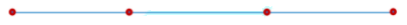When 3 points are collinear. When we join the points in the following manner, we get a triangle.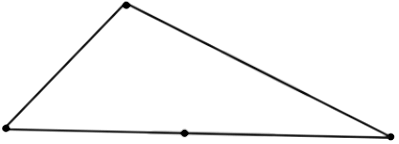No three points out of four are collinear, we obtain a closed figure with four sides. Such a figure formed by joining four points in order is called a quadrilateral..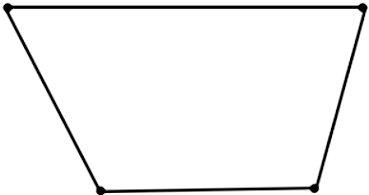A closed, two-dimensional figure formed by four-line segments is called a quadrilateral.
Thus, a plane figure bounded by four-line segments AB, BC, CD, and DA is called a quadrilateral ABCD and is written as quad. ABCD or □ ABCD.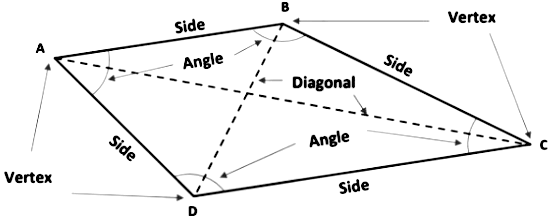• The word quadrilateral has originated from two Latin words, quad which means “four” and, lateral meaning “side”.
• A quadrilateral has four sides (AB, BC, CD, & DA), four angles (∠ ABC, ∠ BCD, ∠ CDA & ∠ DAB), four vertices(A, B, C & D) and two diagonals (AC & BD).

When we look around our surroundings, we find so many objects which are in the shape of a quadrilateral. For example, windows on the wall, the blackboard in your school, the top of the study table, the screen of the computer, the screen of the LCD TV, the screen of the mobile phone, pages in the book, etc. Some of these shapes are

given below.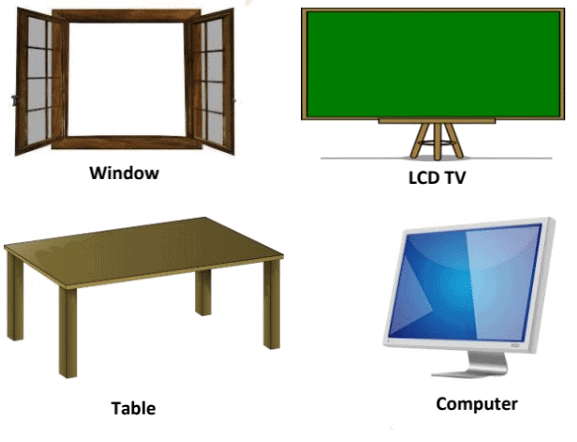Let ABCD is a quadrilateral, which is shown in the following figure.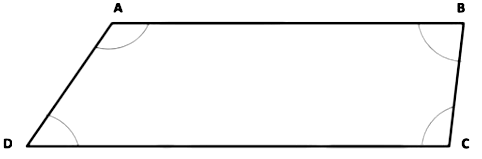• Adjacent sides: Two sides of a quadrilateral are consecutive or adjacent sides if they have a common point (vertex).
AB & BC are adjacent sides with common vertex B; BC & CD are adjacent sides with common vertex C; CD & DA are adjacent sides with common vertex D and DA & AB are adjacent sides with common vertex A.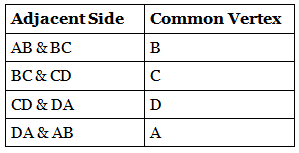• Opposite Sides: Two sides of a quadrilateral are opposite if they have no common endpoint (vertex).
In quadrilateral ABCD, AB & DC are opposite sides. Similarly, BC & AD are also a pair of opposite sides.
• Adjacent Angles: Two angles are adjacent; if they have a common arm.
∠ ABC & ∠ BCD are adjacent angles with common arm BC; ∠ BCD & ∠ CDA are adjacent angles with common arm CD; ∠ CDA & ∠ DAB are adjacent angles with common arm DA and ∠ DAB & ∠ ABC are adjacent angles with common arm AB.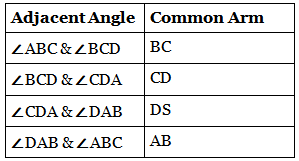• Opposite Angles: Two angles of a quadrilateral are said to be opposite angles if they do not have a common arm. ∠ ABC & ∠ CDA; ∠ BCD & ∠ DAB are two pairs of opposite angles of □ ABCD.

Angle Sum Property of a Quadrilateral
Theorem 1: The sum of the four angles of a quadrilateral is 360°.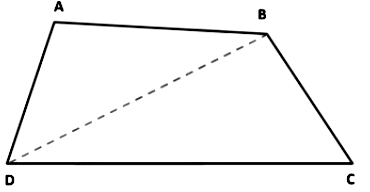Given: ABCD is a quadrilateral
To Prove: ∠ ABC + ∠ BCD + ∠CDA + ∠ DAB = 360°.
Construction: Join BD.
Proof: In ∆ ABD, we have
Since the sum of all angles of a triangle is 180°.
∠ DAB + ∠ ABD + ∠BDA = 180°. ................ (I)
Similarly, in ∆ CBD, we have
∠ DBC + ∠ BCD + ∠CDB = 180°. ................ (II)
Adding equation (I) & (II), we get
∠ DAB + ∠ ABD + ∠BDA + ∠ DBC + ∠ BCD + ∠CDB = 180° + 180°
⟹ ∠ DAB + (∠ ABD + ∠ DBC) + ∠ BCD + (∠CDB + ∠BDA) = 360°.
[∵ ∠ ABD + ∠ DBC = ∠ ABC & ∠CDB + ∠BDA = ∠CDA]
⟹ ∠ DAB + ∠ ABC + ∠ BCD + ∠CDA = 360°.

Example: In a quadrilateral ABCD, the angles A, B, C, and D are in the ratio 2: 3: 1: 4. Find the measure of each angle of the quadrilateral.

Since, angles A, B, C and D are in the ratio 2 : 3 : 1 : 4. For exact values, they contain a common factor x, which we have to find.
Let ∠ DAB = 2x°, ∠ ABC = 3x°, ∠ BCD = x° and ∠CDA = 4x°.
By the angle sum property of quadrilateral,
∴ ∠ DAB + ∠ ABC + ∠ BCD + ∠CDA = 360°.
⟹ 2x° + 3x° + x° + 4x° = 360°.
⟹ 10x° = 360°.
⟹ x° = 360/10°
⟹ x° = 36°.
Thus, the angles are
∠ DAB = 2x° = 2 × 36° = 72°
∠ ABC = 3x° = 3 × 36° = 108°
∠ BCD = x° = 36°.
∠ CDA = 4 × 36° = 144°.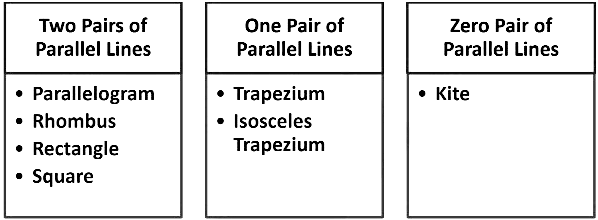1. Parallelogram: A quadrilateral is a parallelogram if its pairs of opposite sides are parallel and equal in length.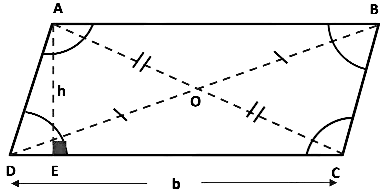Quadrilateral ABCD is a parallelogram because AB ∥ CD and BC ∥ AD.

• Diagonals bisect each other at O. i.e. AO = OC & DO = OB.
• Both pairs of opposite angles are equal. i.e. ∠ ADC = ∠ ABC & ∠ DAB = ∠ BCD.
• Consecutive angles are supplementary (Sum of these angles is 180°). i.e. ∠ ADC + ∠ BCD = 180° & ∠ DAB + ∠ ABC = 180°.

Area of Parallelogram
The area of a parallelogram is equal to the product of its base and its height.
Area = Base × Height
Area = b × h.
Note: Base is the side on which the height (perpendicular) is drawn.
Height is the perpendicular drawn from opposite vertex to its base.

2. Rhombus: A parallelogram having all sides equal is called a rhombus.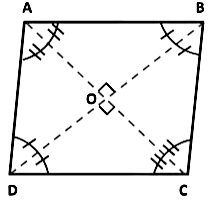Thus, a parallelogram ABCD is a rhombus if AB = AD = BC = CD.

• Diagonals AC & DB are perpendicular to each other. i.e. ∠ AOB = 90° , ∠ COD = 90° , ∠ AOD = 90° and ∠ COB = 90° ,
• Diagonals AC bisects ∠DAB and ∠BCD and diagonal BD bisects ∠ABC and ∠ADC.
∠ ABO = ∠ CBO = ∠ CDO = ∠ ADO & ∠ BAO = ∠ DAO = ∠ BCO = ∠ DCO

Area of Rhombus
The Area of a rhombus is equal to the product of its side and its height.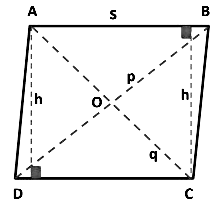Area = side × height
Area = s × h.
Or, the area of a rhombus can also be found out by multiplying the lengths of the diagonals and then divide by 2.
Area = p x q / 2  ; where p and q are diagonals of the rhombus.

3. Rectangle: A parallelogram in which each angle is a right angle and opposite sides are equal.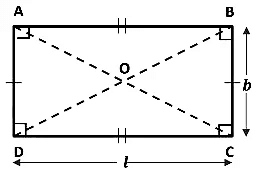Thus, a parallelogram ABCD is a rectangle when AB = CD, BC = AD & ∠ ABC = ∠ BCD = ∠CDA = ∠ DAB = 90°.
In a rectangle, its diagonals are equal. i.e. AC = BD.
And diagonals bisect each other. So, OD = OB and OC = OA.

Area of a Rectangle
The area of the rectangle is equal to the product of its length and its breadth.
Area = l × b.

4. Square: A square is a rectangle with a pair of adjacent sides equal.
In other words, a parallelogram having all sides equal and each angle equal to a right angle is called a square.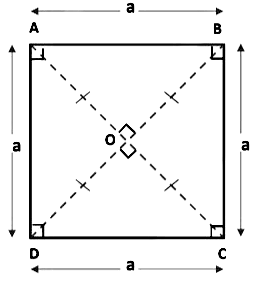Thus, a quadrilateral ABCD is a square in which AB = BC = CD = DA & ∠ ABC = ∠ BCD = ∠CDA = ∠ DAB = 90°.
A square has

• All the properties of a parallelogram.
• All the properties of a rectangle.
• All the properties of a rhombus.

Area of a Square
The Area of square is equal to the square of its side length
Area = side × side
Area = a × a = a2

5. Trapezium: A quadrilateral having exactly one pair of parallel sides is called a trapezium.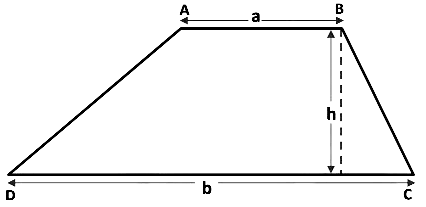ABCD is a trapezium in which AB ∥ CD.
Area of Trapezium
The area of trapezium is equal to the product of the sum of parallel sides and the distance between them.
The parallel sides are called the bases of the trapezium and the distance between the bases is the height1/2 × Sum of parallel sides × Distance between them.
Area = 1/2 × (a + b) × h.

6. Isosceles Trapezium: A trapezium is said to be an isosceles trapezium, if its non-parallel sides are equal.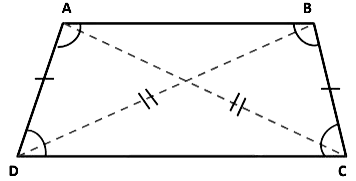Thus, a quadrilateral ABCD is isosceles trapezium in which AB ∥ CD and AD = BC.In an isosceles trapezium

• Its diagonals AC and BD are equal.
• Adjacent angles on non-parallel sides form linear pair of angles. So, ∠ ADC + ∠ DAB = 180° and ∠ BCD + ∠ ABC = 180°.
• Adjacent base angles are equal.
(i) When DC is base then, ∠ ADC = ∠ BCD.
(ii) When AB is base then, ∠ DAB = ∠ ABC.

7. Kite: A quadrilateral is a kite if it has two pairs of equal adjacent sides and unequal opposite sides.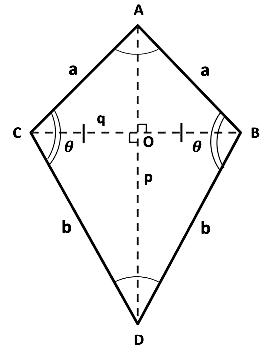• Each pair is made of two adjacent sides that are equal in length.
So, AB = AC and DC = DB.
• The angles are equal where the pairs meet. So, ∠ ACD = ∠ ABD.
• The dotted lines are diagonals, which meet at a right angle.
So, ∠ AOB = ∠ COD = ∠ BOD = ∠ COA = 90°.
• Diagonal AD bisects BC. So, OC = OB.

Area of Kite
The area of a kite is half the product of lengths of its diagonals.
Area = p x q / 2

Properties of Parallelogram
Theorem 2: A diagonal of a parallelogram divides the parallelogram into two congruent triangles.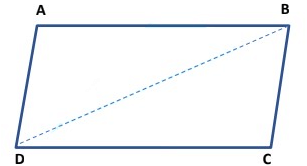Given: ABCD is a parallelogram.
To prove: ∆ ABD ≅ ∆ CDB
Construction: Join BD.
Proof: Since ABCD is a parallelogram. Therefore, AB ∥ DC and BC ∥ AD.
Now, AB ∥ DC and transversal BD intersects them at B and D respectively.
∴ ∠ ABD = ∠ CDB              .................. (I)              [Alternate interior angles]
Again, BC ∥ AD and transversal BD intersects them at B and D respectively.
∴ ∠ ADB = ∠ DBC              .................. (II)            [Alternate interior angles]
Now, in ∆ ABD and ∆ BDC, we have
∠ ABD = ∠ CDB                  [From (I)]
BD = DB                           [Common side]
∠ ADB = ∠ DBC                   [From (II)]
Therefore, ∆ ABD ≅ ∆ CDB (By ASA-criterion of congruence)

Example: In the figure, quadrilateral ABCD is a rectangle in which BD is diagonal. Show that ∆ ABD ≅ ∆ CDB.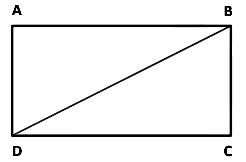Given: ABCD is a rectangle in which BD is diagonal.
To prove: ∆ ABD ≅ ∆ CDB.
Proof: Quadrilateral ABCD is a rectangle. Therefore, ABCD is also a parallelogram.
Since a diagonal of a parallelogram divides it into two congruent triangles.
Hence, ∆ ABD ≅ ∆ CDB.

Theorem 3: In a parallelogram, opposite sides are equal.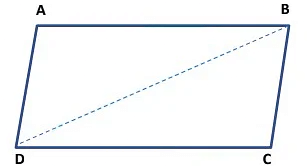Given: ABCD is a parallelogram.
To prove: AB = CD and AD = CB.
Construction: Join BD.
Proof: Since ABCD is a parallelogram. Therefore, AB ∥ DC and BC ∥  AD.
Now, AB ∥ DC and transversal BD intersects them at B and D respectively.
∴ ∠ ABD = ∠ CDB       .................. (I) [Alternate interior angles]
Again, BC ∥ AD and transversal BD intersects them at B and D respectively.
∴ ∠ ADB = ∠ DBC      .................. (II) [Alternate interior angles]
Now, in ∆ ABD and ∆ BDC, we have
∠ ABD = ∠ CDB                             [From (I)]
BD = DB                                      [Common side]
∠ ADB = ∠ DBC                              [From (II)]
Therefore, ∆ ABD ≅ ∆ CDB (By ASA-criterion of congruence)
By using corresponding parts of congruent triangles
⇒ AB = CD and AD = CB.

Theorem 4: If each pair of opposite sides of a quadrilateral is equal, then it is a parallelogram.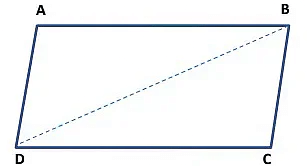Given: A quadrilateral ABCD in which AB = CD and AD = BC
To prove: ABCD is a parallelogram.
Construction: Join BD.
Proof: Now, in ∆ ABD and ∆ CDB, we have
AB = CD                               [Given]
BD = DB                               [Common side]
Therefore, ∆ ABD ≅ ∆ CDB (By SSS-criterion of congruence)
By using corresponding parts of congruent triangles
⟹ ∠ ABD = ∠ CDB                          .......... (I)
⟹ ∠ ADB = ∠ DBC                          .......... (II)
Now, line BD intersects AB and CD at B and D, such that
∠ ABD = ∠ CDB                         [From (I)]
That is, alternate interior angles are equal.
⟹ AB ∥ CD                               .......... (III)
Again, line BD intersects BC and AD at B and D such that.
∠ ADB = ∠ DBC                       [From (II)]
That is, alternate interior angles are equal.
⟹ AB ∥ CD                               .......... (IV)
From (III) and (IV), we have
AB ∥ CD and BC ∥ AD.
Hence, ABCD is a parallelogram.

Example: Find the values of x and y.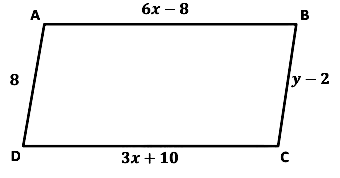Quadrilateral ABCD is a parallelogram. So, opposite sides are equal. Then,
AB = DC
⟹ 6x - 8 = 3x + 10.
⟹ 6x - 3x = 10 + 8.
⟹ 3x = 18.
⟹ x =18.3
∴ x = 6.
⟹ y – 2 = 8
⟹ y = 8 + 2
∴ y = 10.

Theorem 5: In a parallelogram, opposite angles are equal.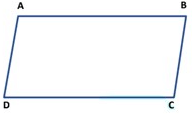Given: ABCD is a parallelogram.
To prove: ∠ DAB = ∠ BCD and ∠ ABC = ∠CDA.
Proof: Since ABCD is a parallelogram. Therefore, AB ∥ CD and BC ∥ AD.
Now, AB ∥ CD and transversal AD intersects them at A and D respectively.
Since the sum of interior angles on the same side of the transversal is 180°.
∴ ∠ DAB + ∠ CDA = 180°                                        ...... (I)
Similarly, BC ∥ AD and transversal CD intersect them at C and D respectively.
∴ ∠ CDA + ∠ BCD = 180°                                        ...... (II)
From (I) and (II), we get
∠ DAB + ∠ CDA = ∠ CDA + ∠ BCD
⟹ ∠ DAB = ∠ BCD.
Now, AD ∥ BC and transversal AB intersect them at A and B respectively.
Since the sum of interior angles on the same side of the transversal is 180°.
∴ ∠ DAB + ∠ ABC = 180°                                   ...... (III)
Similarly, AB ∥ CD and transversal AD intersects them at A and D respectively.
∴  ∠ DAB + ∠ CDA = 180°                                   ...... (IV)
From (III) and (IV), we get
∠ DAB + ∠ ABC = ∠ DAB + ∠ CDA
⟹ ∠ ABC = ∠ CDA.
Similarly,  ∠ ABC = ∠ CDA.
Hence, ∠ DAB = ∠ BCD and ∠ ABC = ∠CDA.

Theorem 6: If in a quadrilateral, each pair of opposite angles is equal, then it is a parallelogram.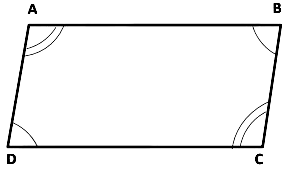Given: A quadrilateral ABCD in which ∠ DAB = ∠ BCD and ∠ ABC = ∠CDA.
To prove: ABCD is a parallelogram.
Proof: In quadrilateral ABCD, we have
∠ DAB = ∠ BCD (Given)                            ......... (I)
⟹ ∠ ABC = ∠CDA (Given)                           ......... (II)
From (I) & (II), we get
∠ DAB + ∠ ABC = ∠ BCD + ∠CDA          .......... (III)
Since, sum of the all interior angles of a quadrilateral is 360°
∴ ∠ DAB + ∠ ABC + ∠ BCD + ∠ CDA = 360° .... (IV)
⟹ ∠ DAB + ∠ ABC +∠ DAB + ∠ ABC = 360° [From (III)]
⟹ 2(∠ DAB + ∠ ABC) = 360°
⟹ ∠ DAB + ∠ ABC = 180°
∴ ∠ DAB + ∠ ABC = ∠ BCD + ∠CDA = 180° .......... (V)
Now, line AB intersects AD and BC at A and B respectively, such that
∠ DAB + ∠ ABC = 180°                              [From (V)]
That is, the sum of interior angles on the same side of the transversal is 180°.
⟹ AD ∥ BC                                                     .......... (VI)
Again, line BC intersects AB and CD at B and C respectively, such that.
∠ BCD + ∠ ABC = 180°               [ ∵ ∠ DAB = ∠ BCD]
That is, the sum of interior angles on the same side of the transversal is 180°.
⟹ AB ∥ CD                                                .......... (VII)
From (VI) and (VII), we have
AB ∥ CD and AD ∥ BC.
Hence, ABCD is a parallelogram.

Example: A parallelogram ABCD is shown in the figure. Find the missing angles.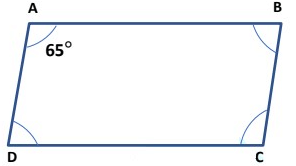Given: ∠ DAB = 65°.
To find: ∠ ABC, ∠ BCD, and ∠CDA.
ABCD is a parallelogram. So, the opposite angles are equal.
∠ BCD = ∠DAB.
∠ BCD = 65°.                                  ............. (I)
And also, adjacent angles are supplementary. We have
∠ BCD + ∠ ABC = 180°
⟹ 65° + ∠ ABC = 180°                       [From (I)]
⟹ ∠ ABC = 180° - 65°
⟹ ∠ ABC = 115°.                              ............ (II)
Since, in a parallelogram, opposite angles are equal.
Therefore,           ∠CDA = ∠ ABC .
⟹                        ∠CDA = 115°.                              [From (II)]
Hence,                ∠ ABC = 115°.
∠ BCD = 65°.
And, ∠CDA = 115°.

Theorem 7: The diagonals of a parallelogram bisect each other.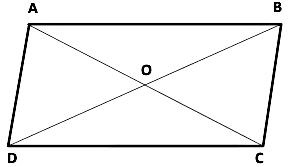Given: ABCD is a parallelogram.
To prove: OA = OC and OD = OB.
Proof: Since ABCD is a parallelogram. Therefore, AB ∥ CD and BC ∥ AD.
Now, AB ∥ CD and transversal BD intersects them at B and D respectively.
∴ ∠ ABD = ∠ CDB                     [Alternate interior angles]
⟹ ∠ ABO = ∠ CDO                                        .................. (I)
Again, AB ∥ CD and transversal AC intersects them at A and C respectively.
∴ ∠ BAC = ∠ DCA                    [Alternate interior angles]
⟹ ∠ BAO = ∠ DCO                                        .................. (I)
Now, in ∆ AOB and ∆ COD, we have
∠ ABO = ∠ CDO                                               [From (I)]
AB = CD                [Opposite side of parallelogram are equal]
∠ BAO = ∠ DCO                                                           [From (II)]
Therefore, ∆ AOB ≅ ∆ COD (By ASA-criterion of congruence)
By using corresponding parts of congruent triangles
⇒ OA = OC and OD = OB.
Hence, the diagonals of a parallelogram bisect each other.

Theorem 8: If the diagonals of a quadrilateral bisect each other, then it is a parallelogram.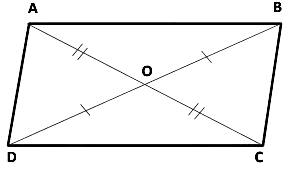Given: A quadrilateral ABCD in which the diagonals AC and BD intersect at O such that OA = OC and OD = OB.
To prove: AB ∥ CD & AD ∥ BC
Proof: In ∆ AOD and ∆ COB, we have
OA = OC                                 (Given)
∠ AOD = ∠ COB                        (Vertically opposite angles)
OD = OB                                 (Given)
Therefore, ∆ AOD ≅ ∆ COB (By SAS-criterion of congruence)
By using corresponding parts of congruent triangles
⇒ ∠ OAD = ∠ OCB                         ............... (I)
Now, line AC intersects BC and AD at C and A respectively, such that
∠ OAD = ∠ OCB                           [From (I)].
That is, alternate interior angles are equal.
∴ AD ∥ BC.                                   ........... (II)
Now, in ∆ AOB and ∆ COD, we have
OB = OD                                      (Given)
∠ AOB = ∠ COD (Vertically opposite angles)
OA = OC                                      (Given)
Therefore, ∆ AOB ≅ ∆ COD                   (By SAS-criterion of congruence)
By using corresponding parts of congruent triangles
⇒ ∠ OBA = ∠ ODC                ............... (III)
Now, line BD intersects AB and DC at B and D respectively, such
that ∠ OBA = ∠ ODC                    [From (III)].
That is, alternate interior angles are equal.
∴ AB ∥ DC.                        .............. (IV)
From equation (II) & (IV), we get
Hence, ABCD is a parallelogram.

Example: Find the length of the following diagonals in the parallelogram ABCD:
I. AC
II. BD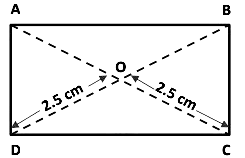Given: OD = OC = 2.5 cm.
To find: Length of AC & BD.
ABCD is a parallelogram. So, the diagonals of parallelogram ABCD bisect each other. Then, BD bisects the AC.
⇒ OA = OC
OA = 2.5 cm.
Then, AC = OA + OC
⇒ AC = 2.5 cm + 2.5 cm.
⇒ AC = 5 cm.
And, AC bisects the BD. Then,
⇒ OD = OB
OD = 2.5 cm.
Then, BD = OD + OB.
⇒ BD = 2.5 cm + 2.5 cm.
⇒ BD = 5 cm.

Another Condition for a Quadrilateral to be a Parallelogram
Theorem 9: A quadrilateral is a parallelogram if a pair of the opposite side is equal and parallel.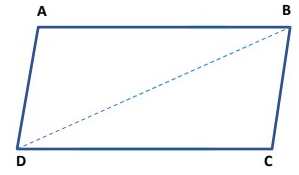Given: A quadrilateral ABCD in which AB = CD and, AB ∥ CD.
To prove: Quadrilateral ABCD is a parallelogram.
Construction: Join BD.
Proof: Now, in ∆ BAD and ∆ DCB, we have
AB = CD                             (Given)
Since AB ∥ CD and transversal BD intersects at B and D, so alternate interior angles are equal.
⟹ ∠ CDB = ∠ ABD
BD = DB                           (Common)
Therefore, ∆ BAD ≅ ∆ DCB             (By SAS-criterion of congruence)
By using corresponding parts of congruent triangles
⟹ ∠ ADB = ∠ CBD
Now, line BD intersects AB and DC at B and D respectively, such that ∠ ADB = ∠ CBD
That is, alternate interior angles are equal.
Thus, AB ∥ CD and AD ∥ BC.
Hence, quadrilateral ABCD is a parallelogram.

Example: In the figure, ABCD is a parallelogram and X, Y are the mid- points of sides AB and DC respectively. Show that quadrilateral DXBY is a parallelogram.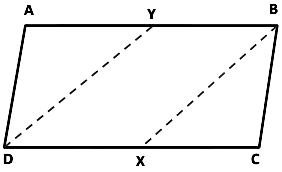Given: ABCD is a parallelogram in which X and Y are the mid-points of AB and DC respectively.
To prove: Quadrilateral DXBY is a parallelogram.
Construction: Join DX and BX.
Proof: Since X and Y are the mid-points of DC and AB respectively.
∴ YB = 1/2 AB and DX = 1/2DC.      ............ (I)
But, AB = DC                   [∵ ABCD is a parallelogram]
⟹ 1/2 AB = 1/2 DC
⟹ YB = DX. [From(I)]                  ............. (II)
Also AB ∥ DC                  [∵ ABCD is a parallelogram]
∴ YB ∥ DX                              ............. (III)
Since a quadrilateral is a parallelogram if a pair of the opposite side is equal and parallel.
From (II) & (III), we get Quadrilateral DXBY is a parallelogram.

The Mid-point Theorem
Theorem 10: The line segment joining the mid-points of two sides of a triangle is parallel to the third side and is equal to half of it.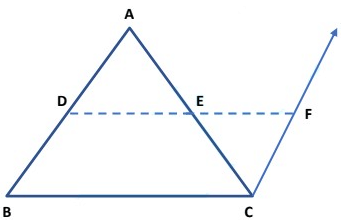Given: A ∆ ABC in which D and E are the mid-points of sides AB and  AC respectively.
To prove: DE ∥ BC and DE = 1/2BC.
Construction: Produce the line segment DE to F, such that DE = EF. Join FC.
Proof: Now, in ∆ AED and ∆ CEF, we have
AE = CE                                  (∵ E is the mid-point of AC)
⟹ ∠ AED = ∠ CEF                         (Vertically opposite angles)
And, DE = FE                                  (By construction)
Therefore, ∆ AED ≅ ∆ CEF                                           (By SAS-criterion of congruence)
By using corresponding parts of congruent triangles
⟹ AD = CF                                      .............. (I)
And, ∠ ADE = ∠ CFE                              .............. (II)
Now, D is the mid-point of AB.
⟹ AD = DB                                      .............. (III)
From equation (I) & (III), we get
CF = DB                                       .............. (IV)
Now, DF intersects AD and FC at D and F respectively such that
∠ ADE = ∠ CFE                                 [From (II)]
That is, alternate interior angles are equal.
⟹ DB ∥ CF.                                         .............. (V)
From (III) & (V), we find that DBCF is a quadrilateral such that one pair of sides is equal and parallel.
∴ DBCF is a parallelogram.
⟹ DF ∥ BC and DF = BC (∵ Opposite sides of a parallelogram are equal and parallel)
But, D, E, F are collinear and DE = EF.
∴ DE ∥ BC and DE = 1/2 BC.

Theorem 11: The line drawn through the mid-point of one side of a triangle, parallel to another side bisects the third side.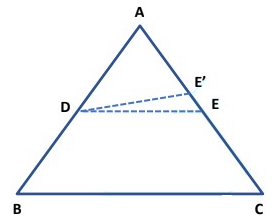Given: ∆ ABC in which D is the mid-point of AB and DE ∥ BC
To prove: E is the mid-point of AC.
Construction: Join DE and DE'.
Proof: We have to prove that E is the mid-point of AC. Suppose, E is not the mid-point of AC. Then, let E' be the mid-point of AC.
Now, in ∆ ABC, D is the mid-point of AB and E' is the mid-point of AC.
But the line drawn through the mid-point of one side of a triangle,  parallel to another side bisects the third side.
Therefore, we have
DE' ∥ BC.                                   ....... (I)
Also Given, DE ∥ BC.                                   ....... (II)
From (I) and (II), we find that two intersecting lines DE and DE' are both parallel to line BC. This is a contradiction.
So, our assumption is wrong. Hence, E is the mid-point of AC.

Example: In the figure, D, E, and F are respectively the mid-points of sides BC, CA, and AB of an equilateral triangle ABC. Prove that triangle DEF is also an equilateral triangle.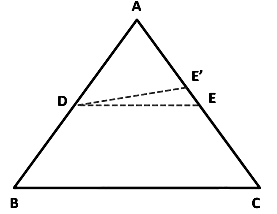Given: D, E, and F are respectively the mid-points of sides BC, CA, and AB of an equilateral triangle ABC.
To prove: DEF is an equilateral triangle.
Construction: Join DE, DF, and FE.
Proof: Since the segment joining the mid-points of two sides of a triangle is half of the third side. Therefore, D and E are the mid-points of BC and AC respectively.
⟹ DE = 1/2 AB                               ............ (I)
E and F are the mid-points of AC and AB respectively.
∴ EF =  1/2  BC.                          ........... (II)
F and D are the mid-points of AB and BC respectively.
∴ FD = 1/2 AC.                     ........... (III)
Now, ∆ ABC is an equilateral triangle.
⟹ AB = BC = CA
⟹ 1/2 AB = 1/2 BC = 1/2 CA
⟹ DE = EF = FD.
Hence, ∆ DEF is an equilateral triangle.

The document Chapter Notes: Quadrilaterals - Notes | Study Mathematics (Maths) Class 9 - Class 9 is a part of the Class 9 Course Mathematics (Maths) Class 9.
All you need of Class 9 at this link: Class 9

## Mathematics (Maths) Class 9

88 videos|397 docs|109 tests
 Use Code STAYHOME200 and get INR 200 additional OFF

## Mathematics (Maths) Class 9

88 videos|397 docs|109 tests

Track your progress, build streaks, highlight & save important lessons and more!

,

,

,

,

,

,

,

,

,

,

,

,

,

,

,

,

,

,

,

,

,

;